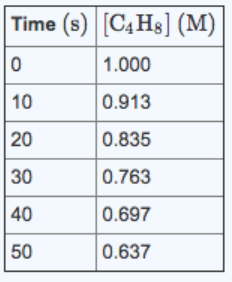# Problem: Consider the following reaction: C4H8 (g) → 2 C2H4 (g) The following data were collected for the concentration of C4H8 as a function of time: A) What is the average rate of the reaction between 0 and 10s?B) What is the average rate of the reaction between 40 and 50s?C) What is the rate of formation of C2H4 between 20 and 30 s?

###### FREE Expert Solution

We’re being asked to calculate the average rate of the reaction for the following reaction:

C4H8 (g) → 2 C2H4 (g)

Recall that for a reaction aA  bB, the rate of a reaction is given by:

$\overline{){\mathbf{Rate}}{\mathbf{=}}{\mathbf{-}}\frac{\mathbf{1}}{\mathbf{a}}\frac{\mathbf{\Delta }\mathbf{\left[}\mathbf{A}\mathbf{\right]}}{\mathbf{\Delta t}}{\mathbf{=}}\frac{\mathbf{1}}{\mathbf{b}}\frac{\mathbf{\Delta }\mathbf{\left[}\mathbf{B}\mathbf{\right]}}{\mathbf{\Delta t}}}$

where:

Δ[A] = change in concentration of reactants or products (in mol/L or M), [A]final – [A]initial

Δt = change in time, tfinal – tinitial

85% (274 ratings)###### Problem Details

Consider the following reaction: C4H8 (g) → 2 C2H4 (g) The following data were collected for the concentration of C4H8 as a function of time:A) What is the average rate of the reaction between 0 and 10s?

B) What is the average rate of the reaction between 40 and 50s?

C) What is the rate of formation of C2H4 between 20 and 30 s?

Frequently Asked Questions

What scientific concept do you need to know in order to solve this problem?

Our tutors have indicated that to solve this problem you will need to apply the Average Rate of Reaction concept. You can view video lessons to learn Average Rate of Reaction. Or if you need more Average Rate of Reaction practice, you can also practice Average Rate of Reaction practice problems.